# Word Problems: Plotting Points

You are familiar with plotting points on a Cartesian plane .

Let us consider the graph.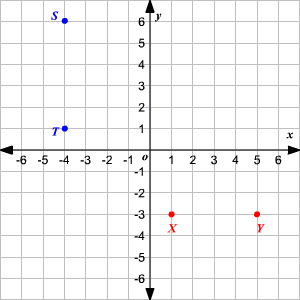What is the distance between the points $X$ and $Y$ ?

First, identify the coordinates of $X$ and $Y$ . The coordinates of $X$ are $\left(1,-3\right)$ and that of $Y$ are $\left(5,-3\right)$ . That is, the two points have the same $y$ -coordinates. So, the distance between the two points is the absolute value of the difference between their $x$ -coordinates. That is, $|\text{\hspace{0.17em}}5-1\text{\hspace{0.17em}}|=4$ .

Now, consider the points $S$ and $T$ . The coordinates of $S$ are $\left(-4,6\right)$ and that of $T$ are $\left(-4,1\right)$ . That is, the two points have the same $x$ -coordinates. So, the distance between the two points is the absolute value of the difference between their $y$ -coordinates. That is, $|\text{\hspace{0.17em}}6-1\text{\hspace{0.17em}}|=5$ .

Now, you can use this strategy to solve some kinds of word problems.

Example 1:

The three points marked are the vertices of a rectangle. Find the fourth vertex.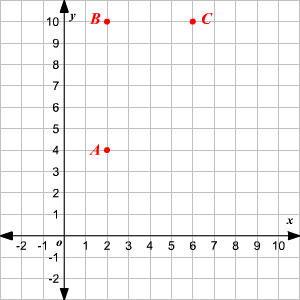The points $A$ and $B$ have the same $x$ -coordinates, so the side $\stackrel{¯}{AB}$ is a vertical line. Also, the points $B$ and $C$ have the same $y$ -coordinates and the side $\stackrel{¯}{BC}$ is a horizontal line. Opposite sides of a rectangle are congruent. The fourth vertex, say $D$ , will have the same $x$ -coordinate as $C$ and the length $\mathrm{AB}$ units away from $C$ . Also, $D$ will have the same $y$ -coordinate as $A$ and the length $\mathrm{BC}$ units away from $A$ . That is, the vertex $D$ will have the coordinates $\left(6,4\right)$ .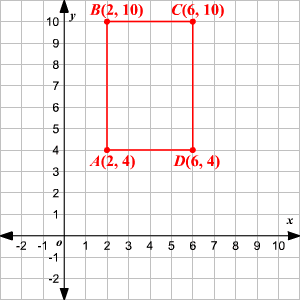Example 2:

The graph shows some places near Isha's home.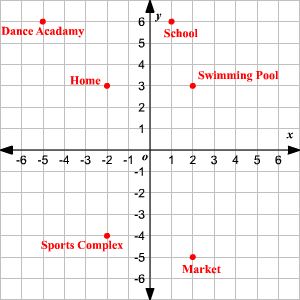a) How far is the sports complex from her home?

b) What is the distance between her home and her swimming pool?

c) How far is the school from the dance academy?

First, let us identify the coordinates of the points that represent each landmark.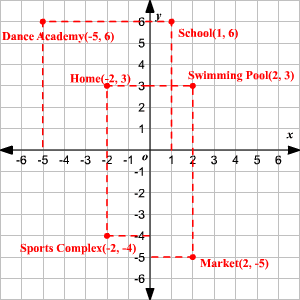a) The sports complex and Isha's home have the same $x$ -coordinates. So, the distance between them is the absolute value of the difference between the $y$ -coordinates.

$|\text{\hspace{0.17em}}-4-3\text{\hspace{0.17em}}|=|\text{\hspace{0.17em}}-7\text{\hspace{0.17em}}|=7$

Therefore, the sports complex is $7$ units away from her home.

b) The home and the swimming pool have the same $y$ -coordinates. So, the distance between them is the absolute value of the difference between the $x$ -coordinates.

$|\text{\hspace{0.17em}}2-\left(-2\right)\text{\hspace{0.17em}}|=|\text{\hspace{0.17em}}4\text{\hspace{0.17em}}|=4$

Therefore, the distance between her home and the swimming pool is $4$ units.

c) The school and the dance academy have the same $y$ -coordinates. So, the distance between them is the absolute value of the difference between the $x$ -coordinates.

$|\text{\hspace{0.17em}}1-\left(-5\right)\text{\hspace{0.17em}}|=|\text{\hspace{0.17em}}6\text{\hspace{0.17em}}|=6$

So, the distance between the school and the dance academy is $6$ units.

Example 3:

The streets of Tiffenville are laid out in a square grid with square city blocks. Joseph and Nisha both start out at the intersection of Idly Street and Puttu Avenue. Joseph walks $4$ blocks north, then $5$ blocks west. Meanwhile Nisha walks $6$ blocks south, then $2$ blocks east, then $10$ blocks north.

If the intersection of Idly Street and Puttu Avenue is considered as the origin, find the coordinates corresponding to Joseph's location.

How far is Joseph away from Nisha?

First, let us consider the paths followed by Joseph and Nisha.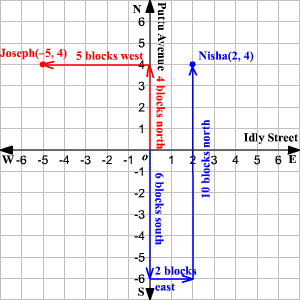The coordinates of the place where Joseph stands are $\left(-5,4\right)$ and that of Nisha are $\left(2,4\right)$ .

The locations of Joseph and Nisha have the same $y$ -coordinates. So, the distance between them is the absolute value of the difference between the $x$ -coordinates.

$|\text{\hspace{0.17em}}\left(2\right)-\left(-5\right)\text{\hspace{0.17em}}|=|\text{\hspace{0.17em}}7\text{\hspace{0.17em}}|=7$

Therefore, Joseph is $7$ blocks away from Nisha.